# National Aeronautics and Space Administration

## Goddard Space Flight Center## How Big Is That Star?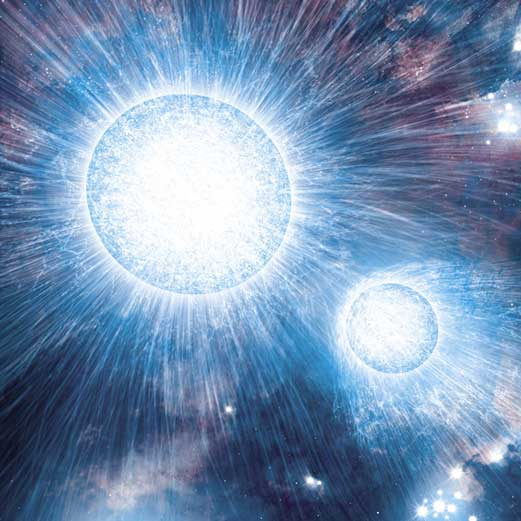# How Big Is That Star?

Estimated class time: 2 class periods

Lesson plan by Kara C. Granger of Maria Carillo High School in Santa Rosa, CA

## Summary

Have you ever wondered about how big stars actually are? Have you ever wondered how astronomers and astrophysicists determine the sizes of stars? Well, everyone can learn how to do this by using the simple representations and manipulating the mathematical equations which are illustrated and demonstrated within this lesson.

## Objectives

1. Students will be able to explain the relationship between radius and mass among a list of stars.
2. Students will understand how a binary star system's orbit can cause changes in the observed brightness of the system.
3. Students will determine the diameters of stars by analyzing data and manipulating equations.

## National Education Standards

### National Science Education Standards

This lesson plan addresses the following National Science Education Standards:

NS.5-8.1 Science as Inquiry
As a result of activities in grades 5-8, all students should develop:

1. Abilities necessary to do scientific inquiry

NS.5-8.5 Science and Technology
As a result of activities in grades 5-8, all students should develop:

1. Understandings about science and technology

NS.9-12.1 Science as Inquiry
As a result of activities in grades 5-8, all students should develop:

1. Abilities necessary to do scientific inquiry

NS.9-12.4 Earth and Space Science
As a result of activities in grades 5-8, all students should develop an understanding of:

1. Origin and evolution of the universe

### National Council of Teachers of Mathematics

This lesson plan addresses the following educational standards from the National Council of Teachers of Mathematics:

• Process Standards
• Problem Solving
Instructional programs from prekindergarten through grade 12 should enable all students to:
1. build new mathematical knowledge through problem solving
2. solve problems that arise in mathematics and in other contexts
• Connections
Instructional programs from prekindergarten through grade 12 should enable all students to:
1. recognize and apply mathematics in contexts outside of mathematics
• Number and Operations
• Understand numbers, ways of representing numbers, relationships among numbers, and number systems
In grades 6-8 all students should:
1. work flexibly with fractions, decimals, and percents to solve problems
2. develop an understanding of large numbers and recognize and appropriately use exponential, scientific, and calculator notation
• Algebra
• Understand patterns, relations, and functions
In grades 6-8 all students should
1. represent, analyze, and generalize a variety of patterns with tables, graphs, words, and, when possible, symbolic rules
• Geometry
• Use visualization, spatial reasoning, and geometric modeling to solve problems
In grades 6-8 all students should
1. recognize and apply geometric ideas and relationships in areas outside the mathematics classroom, such as art, science, and everyday life
• Data Analysis and Probability
• Formulate questions that can be addressed with data and collect, organize and display relevant date to answer them
In grades 6-8 all students should
1. formulate questions, design studies, and collect data about a characteristic shared by two populations or different characteristics within one population
• Select and use appropriate statistical methods to analyze data
In grades 6-8 all students should
1. discuss and understand the correspondence between data sets and their graphical representations, especially histograms, stem-and-leaf plots, box plots, and scatterplots

## Prerequisites

If students have already participated in and completed the Time that Star! lesson, they have a good foundation in astrophysical data and binary star systems. If they have not completed that lesson, then please refer to the following prerequisites.

• Math: Students should have learned some pre-algebraic concepts such pattern recognition, ratios, proportions, decimals, and percents.
• Science: Students should have had an introduction to the concepts of stars and space astronomy.

## Materials

• Teacher
• flash light
• pen light
• two pieces of paper
• red cellophane or filter paper
• Star Dimensions Data Table worksheet (html, Word, PDF)
• Measuring Binary Stars worksheet (html, Word, PDF)
• For each student or pair of students
• calulator
• metric ruler
• a few sheets of graph paper
• handouts

## Guided Practice: Day 1

### Warm-up

Refresh students' memories about ratios by asking a member of the class to define a ratio. Then ask students to write ratios for various things in the classroom: girls to boys, people in the room wearing a red shirt to those with a different color shirt, people in the room writing with a pencil to those writing with a pen, etc. Have them come up with a few of their own, as well. Then have students convert those ratios into decimals and percents.

Now tell students that they will be using ratios to examine the size of some stars in our galaxy. And later they will look at data from binary stars to figure out the sizes of both stars.

### Introducing the size of stars

It is important for students to generally comprehend the size, mass, and density of stars. This introductory activity will establish this general understanding to prepare students for both the lab and the analysis of data from stars within this lesson.

Hand out the Star Dimensions Data Table and Worksheet to students along with star charts (or project a star chart for all students to see). Have students examine the data table complete the worksheet. Here is a summary of the activities on the worksheet:

1. Write a list of the stars from least to greatest in terms of their radius (measured in Suns).
2. Next to each star in the list, write the ratio of radius to mass (measured in Suns).
3. Locate at least four of these stars on a constellation map, sketch the constellation and location of the star.
4. Explain the (very general) relationship between radius and mass among this list of stars.

Also, show students the illustration of the sizes of various stars below. This type of representation should assist visual learners with the concept of the size of stars.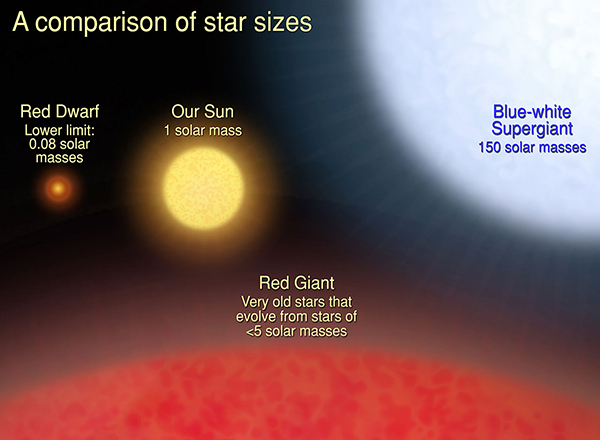Illustration showing the relative sizes of different classes of stars.

### Binary star light curve demo

1. Explain that all types of electromagnetic radiation are emitted in variable amounts from binary systems of stars. Also inform students that high-energy satellites have detected X-rays from these sources, which is the type of data retrieved and used on the second day of this lesson.
2. Next, roll one piece of paper like a tube and tape it around the light end of the flash light. Point it at a blank screen. This demonstrates the "brightness" or "magnitude" of a larger star in a binary system. The paper tube will enable to students to see a well defined circumference around the light.
3. Next, roll one piece of paper like a tube. Tape the red cellophane or filter paper to one end of it. Tape the other end around the light end of the pen light. Be sure the pen light shines through the tube! Now point it at a blank screen. This demonstrates the magnitude of a smaller star in a binary system.
4. Now hold the pen light next to the flash light, pointing both light sources toward a blank wall or screen.
5. Move the pen light so that is "orbits" the flash light. Direct students to notice the change in overall "magnitude" or "brightness" as the pen light is behind, next to, and in front of the flash light. This should be somewhat noticeable since the pen light will show on the wall or screen as red.
6. Prompt the students with questions like, "when is the system brightest?" (when the flash lights are next to each other) and "when is the system dimmest?" (when the pen light is behind the flash light?)
7. Below is a representation of various light curves as they would appear from plotting data from eclipsing binaries. Notice how their "brightness" or "magnitude" changes as the smaller star is behind, next to, and in front of the larger star. Finally, direct students to see how the flash light model and the light curves of binary stars are related. A light curve is simply a plot of some measure of a source's intensity or brightness versus time.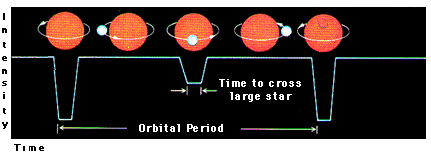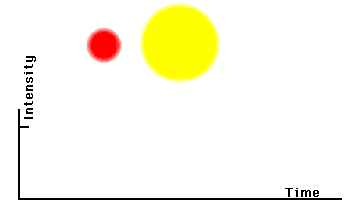Example light curves and models of orbital periods for binary star systems.

### Show me different types of eclipsing binary starsExamples of light curves for different types of binary star systems.

## Guided Practice: Day 2

### Measuring star sizes using binary star light curves

1. Ask students to recall what they did on Day 1. What did they learn about star sizes? What happens in a binary star system when one star is in front of the other? Is it brighter or dimmer than when the stars are next to each other? Etc.

2. Hand out the Measuring Binary Stars Worksheet and have students follow along so they can read and hear the instructions. Next, show students the illustration below. Ask them to describe how the diameter of the smaller star (d1) relates to t1 on the light curve. Then ask them to describe how the larger star (d2) relates to t2 on the light curve.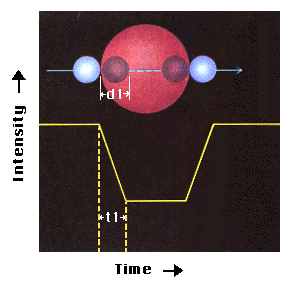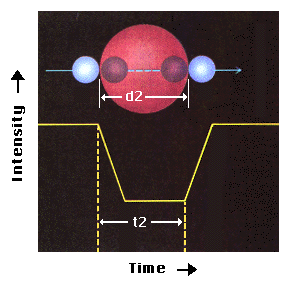Illustration of how the diameter of the stars in an eclipsing binary system relate to dips in the light curve.

In the remainder of this lesson, we will examine data from from sources: HT Cas, X0748-676, and Vela X-1.

### Tell me about HT Cas

HT Cas is a binary system made up of a white dwarf star that is 0.12-times the size of the Sun and a larger star that is 0.154-times the size of the sun.

The system has an inclination of 83 degrees. A system with 90 degrees inclination system would be one where the smaller star traverses the central star exactly in the middle, clike the total eclipse binary type, pictured below.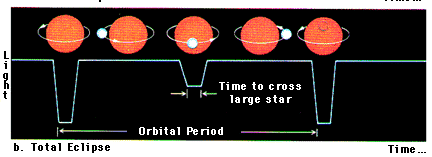Illustration of how the diameter of the stars in an eclipsing binary system relate to dips in the light curve.

From optical observations, astronomers find that the orbital velocity is 390 km/sec.

X0748-676 is a binary star that consists of a smaller star that is a neutron star with an accretion disk and a larger star that is 0.2-times the size of the Sun.

Since this system involves an accretion disk, you won't be able to obtain the size of the neutron star. This is for two reasons. First, the neutron star's accretion disk is never completely eclipsed. And second, the measured d1 would be the diameter of the accretion disk, not the star itself.

Its orbital velocity is 500 km/sec.

### Tell me about Vela X-1

Vela X-1 is a binary system composed of a neutron star in orbit around a B supergiant star that is about 34 times the size of the Sun.

The observed asymmetric light curve is due to enhanced X-ray scattering off of an extended region, which trails the neutron star. This region is created by X-ray ionization of the accreting material.

Its measured orbital velocity is 280 km/sec.

3. Ask your students if they can recall an equation that relates time and distance. Hopefully they will come up with distance = velocity x time.

In the first binary star system example, HT Cas, the velocity is known to be 390 km/sec. Using this velocity, you can now find out the diameter of both stars using the equations d2 = v t2 and d1 = v t1 to determine the diameters of the stars.

Tell me about measuring orbital velocities

To determine the diameter (d2) of the larger star, or the diameter (d1) of the smaller star, we need to look at the light curve, determine both t1 and t2, and calculate.

If we indeed look at the light curve of HT Cas, t1 is equal to 0.4 minutes and t2 is equal to 5.1 minutes. Once we substitute these values, we get:

 d2 = v t2 = 390 km/sec x 4.8 minutes = 390 km/sec x 4.8 x 60 (secs/min.) = 112,320 km d1 = v t1 = 390 km/sec x 0.4 minutes = 390 km/sec x 0.4 x 60 (secs/min.) = 9,360 km

How close are these to the values determined using other data? Using optical data, we obtain values of d2 to be about 214,300 km and d1 about 16,700 km. So we have underestimated both.

The reason is because we are using the x-ray light curve. For d1, did you notice that it was somewhat difficult to determine t1? Astromers' measurements of t1 from this data range from 0.08-0.88 minutes. Also, we are measuring the size of the x-ray emitting region. We find that it is smaller than the size of the white dwarf itself. Both of these contribute to underestimating d1.

For d2 remember that the smaller star does not cross the full diameter of the larger star. This will underestimate the size of the companion star, d2. In addition, studies suggest that the x-ray emitting region is slightly above the plane of orbit of the two stars. This would also cause an additional underestimation of d2.

## Independent Practice

The students are now ready to determine the sizes of the larger stars found in the binary systems X0748-676 and Vela X-1 using the information on their worksheets. The students can work independently or in pairs for the completion of this task.

## Assessment

Formative assessment and observation should be evident throughout the lesson. The worksheet, final questions during closure or a future quiz may serve as summative assessment.

## Closure

Direct students to write for five minutes in their journals summarizing the lab and all procedures in this lesson. Encourage students to then share their findings and what they might have written in their journals.

## References

• Giommi, P., Gottwald, M., Parmar, A. N., and White, N. E., "The Discovery of 3.8 Hour Intensity Dips and Eclipses from the Transient Low-Mass X-ray Binary EXO 0748-676", The Astrophysical Journal, 308:199-212, 1986, September 1.
• Horne, K., Steining, R. F., & Wood, J. H., "Eclipse Studies of the Dwarf Nova HT Cassiopeiae", The Astrophysical Journal, 378:271-280, 1991 September 1.
• Kaufmann, William J. III, Universe, Freeman and Company, 1994, pgs. 336-340
• Kerrod, Robin, Encyclopedia of Science: The Heavens Stars, Galaxies, and the Solar System, Macmillan Publishing Company, 1991
• Kondo, Herbert, The New Book of Popular Science Vol. 1, Grolier Incorporated, 1982, pgs. 174-190
• Seward, Frederick D. and Charles, Philip A., Exploring the X-ray Universe, Cambridge University Press, 1995
• Sato, N., et al., Publications of the Astronomical Society of Japan, 1986, Vol. 38, pg. 731
• Parmar, A. N., et al., Astrophysical Journal, 1991, Vol.366, pg. 253A service of the High Energy Astrophysics Science Archive Research Center (HEASARC), Dr. Alan Smale (Director), within the Astrophysics Science Division (ASD) at NASA/GSFC